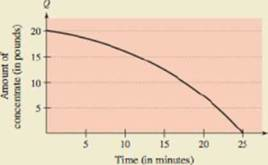Chapter 6.4, Problem 40E

Chapter
Section
Textbook Problem

HOW DO YOU SEE IT? The graph shows the amount of concentrate Q (in pounds) in a solution in a tank at time t (in minute) as a solution with concentrate enters the tank, is well stirred, and is withdrawn from the tank(a) How much concentrate is in the tank at time t = 0 ? (b) Which is greater, the rate of solution into the tank or the rate of solution withdrawn from the tank? Explain.(c) At what time is there no concentrate in the tank? What does this mean?

a)

To determine

To Calculate: The amount of concentrate in the tank at time t=0.

Explanation

Given:

The graph shows the amount of concentrate Q(in pounds) in a solution in tank at time t(in minutes) as a solution with concentrate enters the tank, is well stirred, and is withdrawn from the tank.

Calculation:

b)

To determine

Which is greater, rate of solution into tank or rate of solution withdrawn from tank?

c)

To determine

To Calculate: The time taken to withdraw the amount of concentrate from the tank.

Still sussing out bartleby?

Check out a sample textbook solution.

See a sample solution

The Solution to Your Study Problems

Bartleby provides explanations to thousands of textbook problems written by our experts, many with advanced degrees!

Get Started

Evaluate the expression sin Exercises 116. 23

Finite Mathematics and Applied Calculus (MindTap Course List)

In Exercises 2340, find the indicated limit. 40. limx3xx2+72x2x+3

Applied Calculus for the Managerial, Life, and Social Sciences: A Brief Approach

In Problems 7-34, perform the indicated operations and simplify. 18.

Mathematical Applications for the Management, Life, and Social Sciences

Find each product. 5x4(2x3+8x21)

Elementary Technical Mathematics

Find mRST if mRT:mTS=1:4

Elementary Geometry for College Students

True or False: The vector function is continuous at t = 3.

Study Guide for Stewart's Multivariable Calculus, 8th

cosh 0 = a) 0 b) 1 c) e22 d) is not defined

Study Guide for Stewart's Single Variable Calculus: Early Transcendentals, 8th